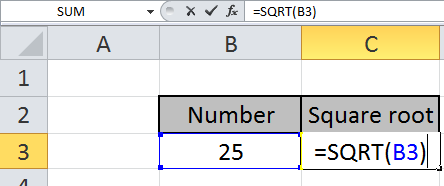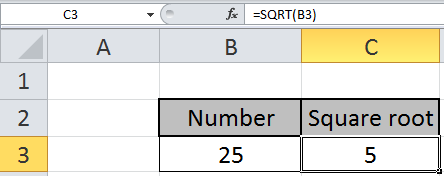# How to use the SQRT Function in Excel

In this article, we will find out how to calculate the square root  of a number using the SQRT function in excel.
SQRT function is used when we need to find the square root of a number in Excel.

The square root of x is referred to as x.

The Root of a number is inverse of Power of the number.

Square of a number = Power(2) of a number

The square root of a number = power(½) of a number

 y = x2  (y)1/2 = x

Syntax of SQRT:

=SQRT(number)

Number : input number you want the square root of.
Let’s look at the example shown here
Use the formula to get the square root of the number in cell B3.

=SQRT(B3)

B3 : input number as a cell reference.Press Enter to see the square root of 25.The square root of 25 is 5.

You can customize your formula with these basic functions. As you can see by using the mathematical function in Excel you can perform many exponents function. Please do find more articles on the mathematical formulation here.

Notes:

1. The function returns the #VALUE! Error, if the argument to the function is non numeric.
2. The function returns the #NUM! Error, if the argument to the function is less than zero. Use the ABS function to the input number to get the square root of a positive number.

Hope you understood how to use SQRT function and referring cell in Excel. Explore more articles on Excel mathematical and trigonometric functions here. Please feel free to state your query or feedback for the above article.

Related Articles

How to use the POWER Function in Excel

Find the nth root of a number

How to use the IMCONJUGATE Function in Excel

How to use the IMEXP Function in Excel

How to use the IMSIN Function in Excel

How to use the IMSUM Function in Excel

How to use the IMSUB Function in Excel

How to use the IMARGUMENT Function in Excel

How to use the IMCOS Function in Excel

Popular Articles:

50 Excel Shortcuts to Increase Your Productivity

How to use the VLOOKUP Function in Excel

How to use the COUNTIF in Excel 2016

Edit a dropdown list

If with conditional formatting

If with wildcards

Vlookup by date

Terms and Conditions of use

The applications/code on this site are distributed as is and without warranties or liability. In no event shall the owner of the copyrights, or the authors of the applications/code be liable for any loss of profit, any problems or any damage resulting from the use or evaluation of the applications/code.